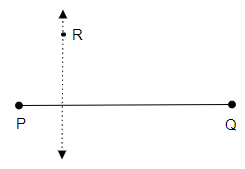# Draw any line segment $\overline{\mathrm{PQ}}$. Take any point $\mathrm{R}$ not on it. Through $\mathrm{R}$, draw a perpendicular to $\overline{\mathrm{PQ}}$. (use ruler and set-square)

To do:

We have to draw a perpendicular to $\overline{PQ}$.

Solution:Steps of construction:

(i) Let us draw a line segment $\overline{PQ}$ and mark a point R outside it.

(ii) Now, place a set square in such a way that one of its right angles aligns with $\overline{PQ}$

(iii) Now, by placing a ruler along the edge opposite to the right angle of the set-square hold the ruler fixed.

(iv) Then, let us slide the set-square along the ruler such that the point R touches the other side of the set-square.

(v) Now, let's draw a line along the edge of the set-square which passes through point R.

(vi) Therefore, the required line perpendicular to $\overline{PQ}$ is formed.

Updated on: 10-Oct-2022

47 Views